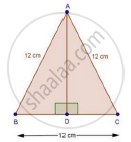Share

# Calculate the Height of an Equilateral Triangle Each of Whose Sides Measures 12 Cm. - Mathematics

Course

#### Question

Calculate the height of an equilateral triangle each of whose sides measures 12 cm.

#### SolutionWe have,

ΔABC is an equilateral Δ with side 12 cm.

Draw AE ⊥ BC

In ΔABD and ΔACD

AB = AC [Each 12 cm]

Then, ΔABD ≅ ΔACD [By RHS condition]

∴ AD2 + BD2 = AB2

⇒ AD2 + 62 = 122

⇒ AD2 = 144 − 36 = 108

⇒ AD = sqrt108 = 10.39 cm

Is there an error in this question or solution?

#### APPEARS IN

RD Sharma Solution for Class 10 Maths (2018 (Latest))
Chapter 7: Triangles
Ex. 7.7 | Q: 16 | Page no. 120
RD Sharma Solution for Class 10 Maths (2018 (Latest))
Chapter 7: Triangles
Ex. 7.7 | Q: 16 | Page no. 120

#### Video TutorialsVIEW ALL 

Solution Calculate the Height of an Equilateral Triangle Each of Whose Sides Measures 12 Cm. Concept: Application of Pythagoras Theorem in Acute Angle and Obtuse Angle.
S